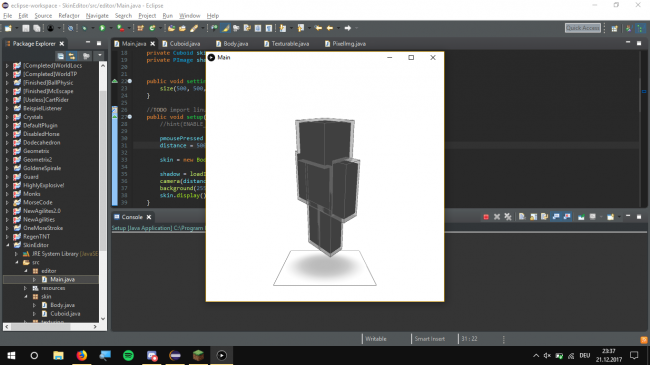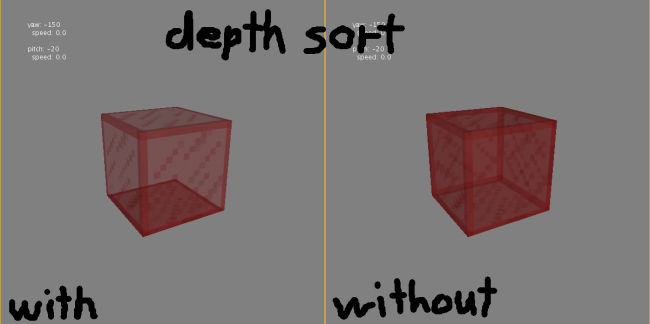#### Howdy, Stranger!

We are about to switch to a new forum software. Until then we have removed the registration on this forum.

# enabling depth sort causes extreme lag...

edited December 2017

Hey guys, I am currently trying to make a mc skin editor (yeah kill me). I know that processing is just for small sketchest but I am srsly to lazy to learn anything about OpenGL. Anyway, I will porbably use some transparent elements and therefore it would be noice to have a hint(ENABLE_DEPTH_SORT);The thing is that I am hardly getting 2fps when I use depth sort. I mean I did not imagine 6x6=36 squares with opacity to slow down the program so badly...

Ik I did not post any code here but is there anything I should know about the depth sort thing or does anybody know anything about this problem?

Tagged:

• edited December 2017 Answer ✓

you should post an MCVE anyway.

``````void setup() {
size(920, 540, P3D);
noStroke();
}
float angle=0.;
void draw() {

//
// hint(ENABLE_DEPTH_SORT);

background(255);
directionalLight(51, 102, 126, 0, 0, -1);

// cubes semi transparent
for (int i = 0; i < 100; i++) {
pushMatrix();
fill(255, 255, 255, 155);
translate(width/2, (i*50), -50*i );
rotateX(angle);
rotateY(angle);
box(50);
popMatrix();
}

// red cube
blendMode(NORMAL);
pushMatrix();
fill(255, 0, 0, 255);
translate(width/2, height/2);
rotateY(angle);
rotateX(angle);
box(100);
popMatrix();

angle=frameCount*.01f;

if (frameCount % 30 == 0) println(frameRate);
}
``````
• @nabr mhhh... I forgot about some spheres I placed in the middle of each block. since they are made of a whole lot of triangles they may cause problems in combination with depth sort...

• Look at sphereDetail in the reference for the spheres

• General question: what’s the purpose of hint(ENABLE_DEPTH_SORT);? I thought P3D would sort the objects correctly in 3D space by default anyway?

• edited December 2017

@Chrisir well if you work with opacity just drawing the top elements is not enough, some have to be drawn first and some lateri think this should show it nicely, just the other way around xD

• edited December 2017 Answer ✓

@someDude

Okay, i understand now, i out of time "on vacation".

Make sure you optimize your code.

Here is the Low Level implementation of Alpha Blending without ENABLE_DEPTH_SORT (i already was preparing, and then you posted your pics)

Maybe you find a tutorial, and can fix it be your self. Would be the best :)
like here http://www.alecjacobson.com/weblog/?p=2750

``````import com.jogamp.opengl.GL;
import com.jogamp.opengl.GL2ES2;
PShape s;

PJOGL pgl;
GL2ES2 gl;

void setup() {
size(856, 580, P3D);
//50.000 verts  ~3.3Mb
s.scale(15);
// s.rotateX(PI);
}

void draw() {
pgl = (PJOGL) beginPGL();
gl = pgl.gl.getGL2ES2();

gl.glClearDepth( 1.0f );
gl.glEnable( GL.GL_DEPTH_TEST );
gl.glDepthFunc( GL.GL_LEQUAL );

// background(0);
// JOGL equivalent
gl.glClearColor(0.0f, 0.0f, 0.0f, 1.0f);
gl.glClear(GL.GL_COLOR_BUFFER_BIT | GL.GL_DEPTH_BUFFER_BIT);

directionalLight( 253, 184, 19, 0, 0, -1);

// JOGL equivalent
gl.glEnable(GL.GL_CULL_FACE ); // for obj - works with processing primitives
// BACK_FACE (default)
gl.glEnable(GL.GL_BLEND);
gl.glBlendFunc(GL.GL_SRC_ALPHA, GL.GL_ONE);

translate(width*.5f, height*.5f);
rotateY(frameCount*.01f);

for (float i = 0.; i <=TWO_PI; i += PI/20.0) {
pushMatrix();
translate(cos(i)*250.f, sin(i)*250.f);
rotateX(frameCount*.01f);
shape(s);
popMatrix();
}
if (frameCount % 30 == 0) println(frameRate);

endPGL();
}
``````